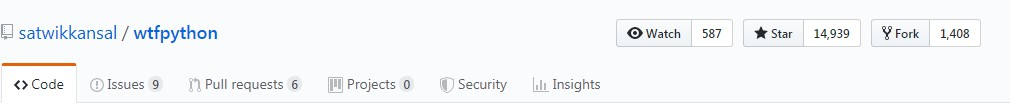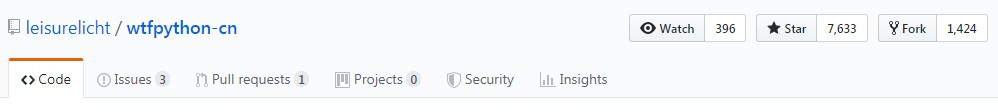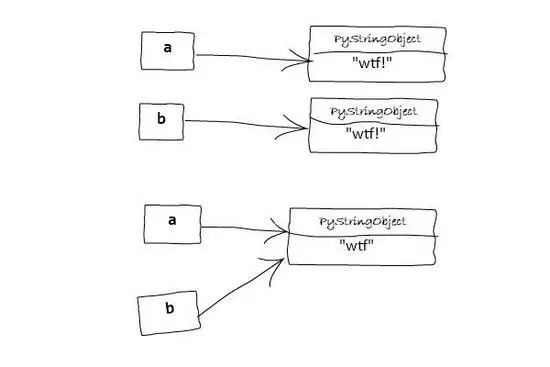# Python 的 51 個秘密曝光，Github 獲 2 萬星

Python, 是一個設計優美的解釋型高級語言, 它提供了很多能讓程式員感到舒適的功能特性. 但有的時候, Python 的一些輸出結果對於初學者來說似乎並不是那麼一目瞭然.• 仔細閱讀設置例子最開始的代碼. 如果您是一位經驗豐富的 Python 程式員, 那麼大多數時候您都能成功預期到後面的結果.
• 閱讀輸出結果,
• 如果不知道, 深呼吸然後閱讀說明 (如果你還是看不明白, 別沉默! 可以在這提個 issue).
• 如果知道, 給自己點獎勵, 然後去看下一個例子.
• 確認結果是否如你所料.
• 確認你是否知道這背後的原理.

PS: 你也可以在命令列閱讀 WTFpython. 我們有 pypi 包 和 npm 包(支持代碼高亮).(譯: 這兩個都是英文版的)

Strings can be tricky sometimes/微妙的字串

1、

``````>>> a = "some_string"
>>> id(a)
140420665652016
>>> id("some" + "_" + "string") # 註意兩個的id值是相同的.
140420665652016``````

2、

``````>>> a = "wtf"
>>> b = "wtf"
>>> a is b
True

>>> a = "wtf!"
>>> b = "wtf!"
>>> a is b
False

>>> a, b = "wtf!", "wtf!"
>>> a is b # 僅適用於3.7版本以下, 3.7以後的傳回結果為False.
True``````

3、

``````>>> 'a' * 20 is 'aaaaaaaaaaaaaaaaaaaa'
True
>>> 'a' * 21 is 'aaaaaaaaaaaaaaaaaaaaa'
False``````說明:

• 這些行為是由於 Cpython 在編譯優化時, 某些情況下會嘗試使用已經存在的不可變物件而不是每次都創建一個新物件. (這種行為被稱作字串的駐留[string interning])

• 發生駐留之後, 許多變數可能指向記憶體中的相同字串物件. (從而節省記憶體)

• 在上面的代碼中, 字串是隱式駐留的. 何時發生隱式駐留則取決於具體的實現. 這裡有一些方法可以用來猜測字串是否會被駐留:

• 所有長度為 0 和長度為 1 的字串都被駐留.

• 字串在編譯時被實現 (`'wtf'` 將被駐留, 但是 `''.join(['w', 't', 'f']` 將不會被駐留)

• 字串中只包含字母，數字或下劃線時將會駐留. 所以 `'wtf!'` 由於包含 `!` 而未被駐留. 可以在這裡找到 CPython 對此規則的實現.• 當在同一行將 `a` 和 `b` 的值設置為 `"wtf!"` 的時候, Python 解釋器會創建一個新物件, 然後同時取用第二個變數(譯: 僅適用於3.7以下, 詳細情況請看這裡). 如果你在不同的行上進行賦值操作, 它就不會“知道”已經有一個 `wtf！` 物件 (因為 `"wtf!"` 不是按照上面提到的方式被隱式駐留的). 它是一種編譯器優化, 特別適用於交互式環境.

• 常量摺疊(constant folding) 是 Python 中的一種 窺孔優化(peephole optimization) 技術. 這意味著在編譯時運算式 `'a'*20`會被替換為 `'aaaaaaaaaaaaaaaaaaaa'` 以減少運行時的時鐘周期. 只有長度小於 20 的字串才會發生常量摺疊. (為啥? 想象一下由於運算式 `'a'*10**10` 而生成的 `.pyc` 檔案的大小). 相關的原始碼實現

https://github.com/python/cpython/blob/3.6/Python/peephole.c#L288

### Time for some hash brownies!/是時候來點蛋糕了!

• hash brownie指一種含有大麻成分的蛋糕, 所以這裡是句雙關

1、

``````some_dict = {}
some_dict[5.5] = "Ruby"
some_dict[5.0] = "JavaScript"
some_dict = "Python"``````

Output:

``````>>> some_dict[5.5]
"Ruby"
>>> some_dict[5.0]
"Python"
>>> some_dict
"Python"``````

“Python” 消除了 “JavaScript” 的存在?說明:

• Python 字典通過檢查鍵值是否相等和比較哈希值來確定兩個鍵是否相同.
• 具有相同值的不可變物件在Python中始終具有相同的哈希值.
``````>>> 5 == 5.0
True
>>> hash(5) == hash(5.0)
True``````
• 註意: 具有不同值的物件也可能具有相同的哈希值（哈希衝突）.
• 當執行 `some_dict = "Python"` 陳述句時, 因為Python將 `5` 和 `5.0` 識別為 `some_dict` 的同一個鍵, 所以已有值 “JavaScript” 就被 “Python” 改寫了.
• 這個 StackOverflow的 回答 漂亮的解釋了這背後的基本原理.

https://stackoverflow.com/questions/32209155/why-can-a-floating-point-dictionary-key-overwrite-an-integer-key-with-the-same-v

### Return return everywhere!/到處傳回！

``````def some_func():
try:
return 'from_try'
finally:
return 'from_finally' ``````

Output:

``````>>> some_func()
'from_finally'``````

####說明:

• 當在 “try…finally” 陳述句的 `try` 中執行 `return``break` 或 `continue` 後, `finally` 子句依然會執行.

• 函式的傳回值由最後執行的 `return` 陳述句決定. 由於 `finally` 子句一定會執行, 所以 `finally` 子句中的 `return` 將始終是最後執行的陳述句.

### Deep down, we’re all the same./本質上,我們都一樣.

``````class WTF:
pass``````

Output:

``````>>> WTF() == WTF() # 兩個不同的物件應該不相等
False
>>> WTF() is WTF() # 也不相同
False
>>> hash(WTF()) == hash(WTF()) # 哈希值也應該不同
True
>>> id(WTF()) == id(WTF())
True``````

####說明:

• 當呼叫 `id` 函式時, Python 創建了一個 `WTF` 類的物件並傳給 `id` 函式. 然後 `id` 函式獲取其id值 (也就是記憶體地址), 然後丟棄該物件. 該物件就被銷毀了.

• 當我們連續兩次進行這個操作時, Python會將相同的記憶體地址分配給第二個物件. 因為 (在CPython中) `id` 函式使用物件的記憶體地址作為物件的id值, 所以兩個物件的id值是相同的.

• 綜上, 物件的id值僅僅在物件的生命周期內唯一. 在物件被銷毀之後, 或被創建之前, 其他物件可以具有相同的id值.

• 那為什麼 `is` 操作的結果為 `False` 呢? 讓我們看看這段代碼.

``````class WTF(object):
def __init__(self): print("I")
def __del__(self): print("D")``````

Output:

``````>>> WTF() is WTF()
I
I
D
D
False
>>> id(WTF()) == id(WTF())
I
D
I
D
True``````

正如你所看到的, 物件銷毀的順序是造成所有不同之處的原因.

### For what?/為什麼?

``````some_string = "wtf"
some_dict = {}
for i, some_dict[i] in enumerate(some_string):
pass``````

Output:

``````>>> some_dict # 創建了索引字典.
{0: 'w', 1: 't', 2: 'f'}``````

####說明:

• Python 語法 中對 `for` 的定義是:

``for_stmt: 'for' exprlist 'in' testlist ':' suite ['else' ':' suite]``

其中 `exprlist` 指分配標的. 這意味著對可迭代物件中的每一項都會執行類似 `{exprlist} = {next_value}` 的操作.

一個有趣的例子說明瞭這一點:

``````for i in range(4):
print(i)
i = 10``````

Output:

``````0
1
2
3``````

你可曾覺得這個迴圈只會運行一次?說明:

• 由於迴圈在Python中工作方式, 賦值陳述句 `i = 10` 並不會影響迭代迴圈, 在每次迭代開始之前, 迭代器(這裡指 `range(4)`) 生成的下一個元素就被解包並賦值給標的串列的變數(這裡指 `i`)了.
• 在每一次的迭代中, `enumerate(some_string)` 函式就生成一個新值 `i` (計數器增加) 並從 `some_string` 中獲取一個字符. 然後將字典 `some_dict` 鍵 `i` (剛剛分配的) 的值設為該字符. 本例中迴圈的展開可以簡化為:

``````>>> i, some_dict[i] = (0, 'w')
>>> i, some_dict[i] = (1, 't')
>>> i, some_dict[i] = (2, 'f')
>>> some_dict``````

### Evaluation time discrepancy/執行時機差異

1、

``````array = [1, 8, 15]
g = (x for x in array if array.count(x) > 0)
array = [2, 8, 22]``````

Output:

``````>>> print(list(g))
``````

2、

``````array_1 = [1,2,3,4]
g1 = (x for x in array_1)
array_1 = [1,2,3,4,5]

array_2 = [1,2,3,4]
g2 = (x for x in array_2)
array_2[:] = [1,2,3,4,5]``````

Output:

``````>>> print(list(g1))
[1,2,3,4]

>>> print(list(g2))
[1,2,3,4,5]``````說明:

• 在生成器運算式中, `in` 子句在宣告時執行, 而條件子句則是在運行時執行.

• 所以在運行前, `array` 已經被重新賦值為 `[2, 8, 22]`, 因此對於之前的 `1``8` 和 `15`, 只有 `count(8)` 的結果是大於 `0`的, 所以生成器只會生成 `8`.

• 第二部分中 `g1` 和 `g2` 的輸出差異則是由於變數 `array_1` 和 `array_2` 被重新賦值的方式導致的.

• 在第一種情況下, `array_1` 被系結到新物件 `[1,2,3,4,5]`, 因為 `in` 子句是在宣告時被執行的， 所以它仍然取用舊物件 `[1,2,3,4]`(並沒有被銷毀).

• 在第二種情況下, 對 `array_2` 的切片賦值將相同的舊物件 `[1,2,3,4]` 原地更新為 `[1,2,3,4,5]`. 因此 `g2` 和 `array_2` 仍然取用同一個物件(這個物件現在已經更新為 `[1,2,3,4,5]`).